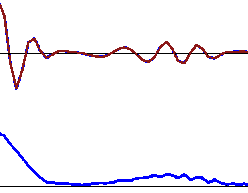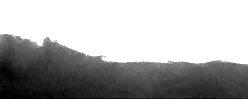## Tuesday, May 26, 2009

### AWT and particle model of quantum mechanics

By AWT Schrödinger equation describes elastic string (red line on the animation bellow), the mass density of which (blue line on the animation bellow) is proportional to energy density of string in each space/time interval (i.e. the Hamiltonian distribution) by mass-energy equivalence principle. Therefore many quantum phenomena can be illustrated by nonlinear elastic liquids, the supercritical fluid at multiple Lifshitz point or soap foam in particular. The inertia and elastic properties of water are subtle, but we can still observe macroscopic analogy of double slit experiment and quantum mechanic behavior at the case of tiny droplets, bouncing on undulating water surface. The direct connection of Newtonian mechanics to quantum mechanics (i.e. a correspondence principle) can be illustrated with computer simulations of elastic fluid composed of repulsing particles. Computing power of contemporary GPU's and parallel processors is sufficient to run such simulations of 300.000+ particles in real time.On the simulation example bellow the density scale of resulting density fluctuations is exaggerated - so we can see clearly, how underwater density wave follows surface waves like so called probability function solution of time dependent Schrödinger equation. The underwater sound wave corresponds the gravitational wave in vacuum analogy, whereas the transversal surface wave corresponds the photon wave. We can see, the underwater wave spreads in much higher speed, then the surface waves, because of higher number of dimensions involved and they're behaving like dispersive tachyons of negative rest mass. The longitudinal character of underwater wave results in fast dispersion, though, so that such waves can mediate only short distance range interactions (Casimir force and weak nuclear force in particular).The density fluctuations of underwater waves correspond wave packets at the water surface, where energy spreads in lower speed, so that the probability of surface waves occurrence increases here. In this way, underwater density fluctuations can model the probability function of quantum wave and as such they can serve as a model of photons. We can see, density of water layers increases with thickness, i.e. with potential energy content and it depends on kinetic energy of surface wave (i.e. the frequency) as well, i.e. by the same way, like Hamiltonian term of Schrödinger equation.At the real situation, the density fluctuations of water are subtle, so that only surface wave can be observed here. Under relativistic situation, when we are forced to observe the water by its own waves exclusively (which is situation, analogous to observation in vacuum), then the surface waves would be unobservable for us via surface waves due the Bose-Einstein distribution of waves, which are penetrating mutually like ghosts (compare the picture above). So that everything, what we would be allowed to see are just the nondispersive wave packets, i.e. solitons, refracting and bouncing from inner walls of underwater density fluctuations like tiny gravitational lenses (wave particle duality). The actual state of surface waves would remain unachievable for us, from this the indeterministic and probabilistic character of quantum mechanics follows.

These connections are all quite simple and trivial - so that only deep misunderstanding of Michelson-Morley experiment and strong disbelief in Aether model has prohibited the mainstream science in recognition of emergent character of quantum mechanics before many years already.